See Temple’s coronavirus response.

# Cost of Equity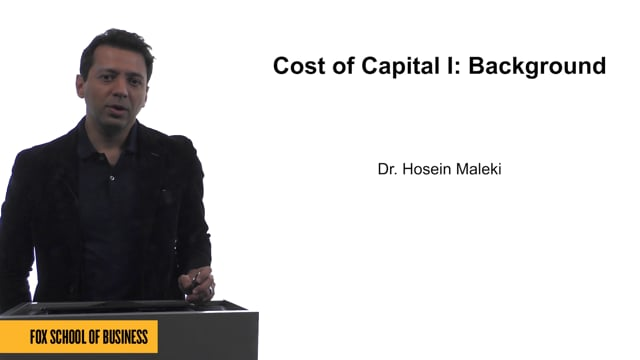### Cost of Capital I: Background

Total Running Time: 04:37
Cost of Capital I: Background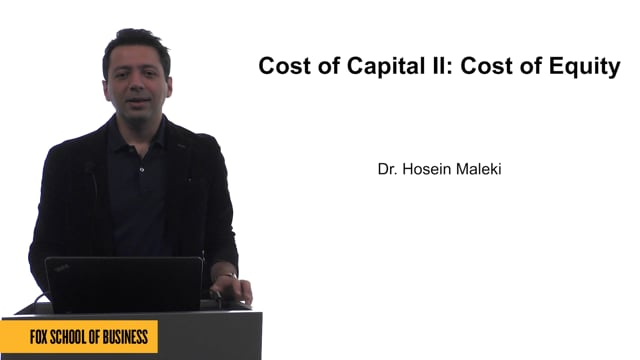### Cost of Capital II: Cost of Equity

Total Running Time: 09:59
Cost of Capital II: Cost of Equity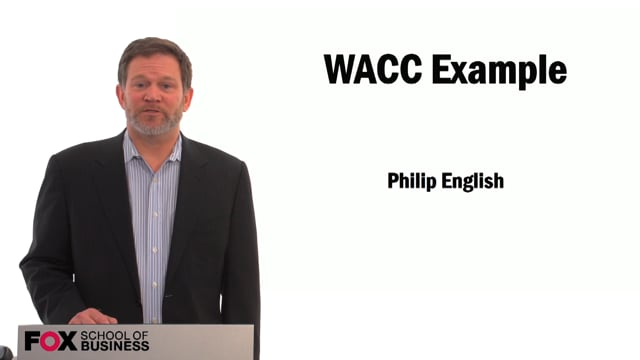### WACC Example

Total Running Time: 06:13
WACC Example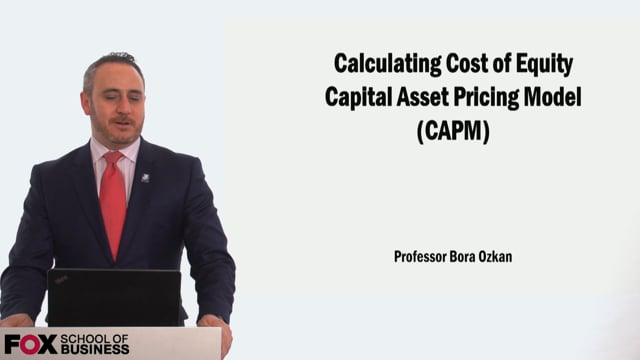### Calculating the Cost of Equity using Capital Asset Pricing Model

Total Running Time: 11:32
Calculating the Cost of Equity using Capital Asset Pricing Model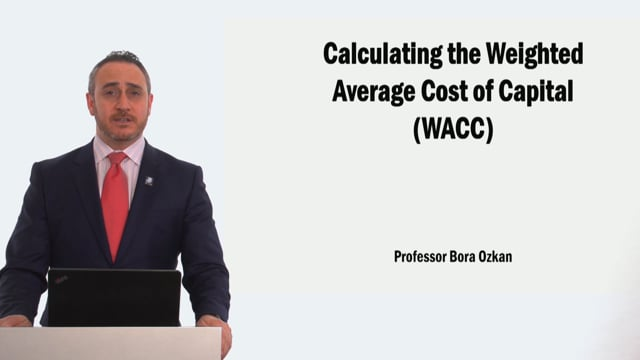### Calculating the Weighted Average Cost of Capital

Total Running Time: 18:43
Calculating the Weighted Average Cost of Capital
Go Back to the top of the pageFox Video Vault vault.temple.edu